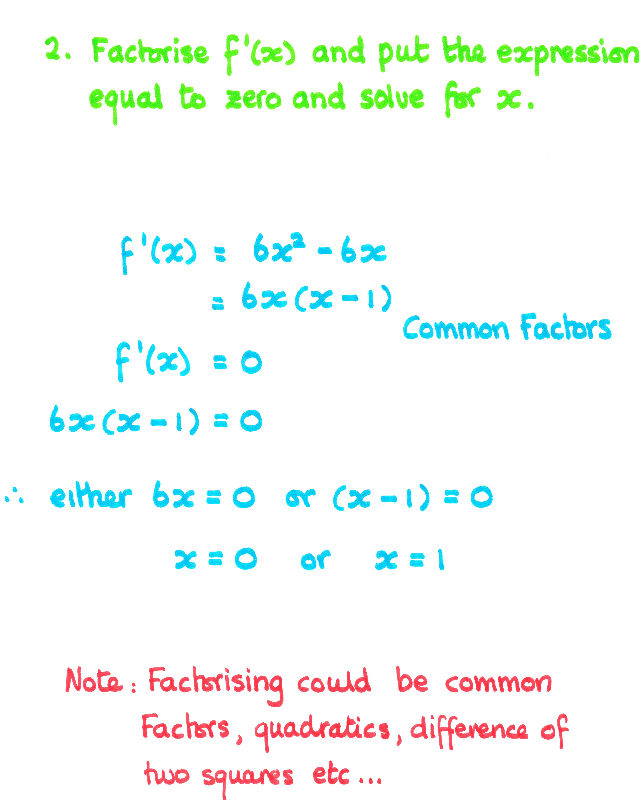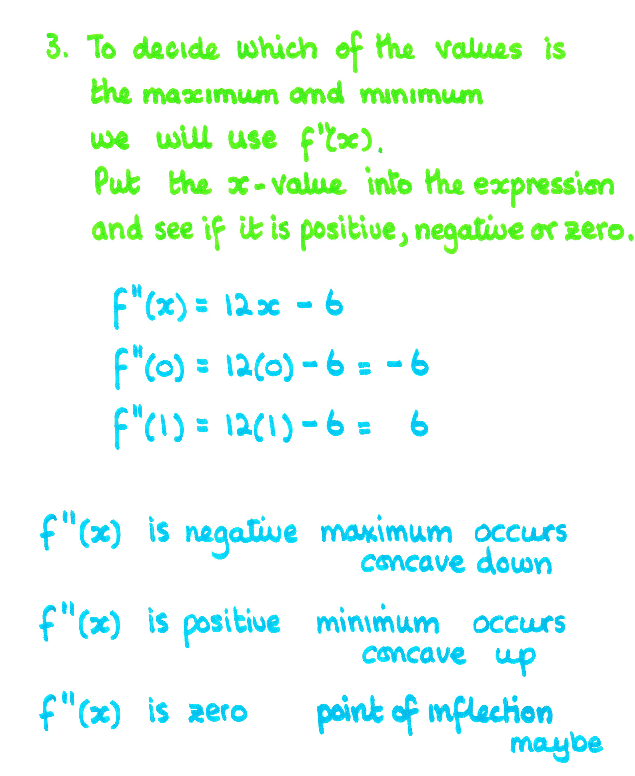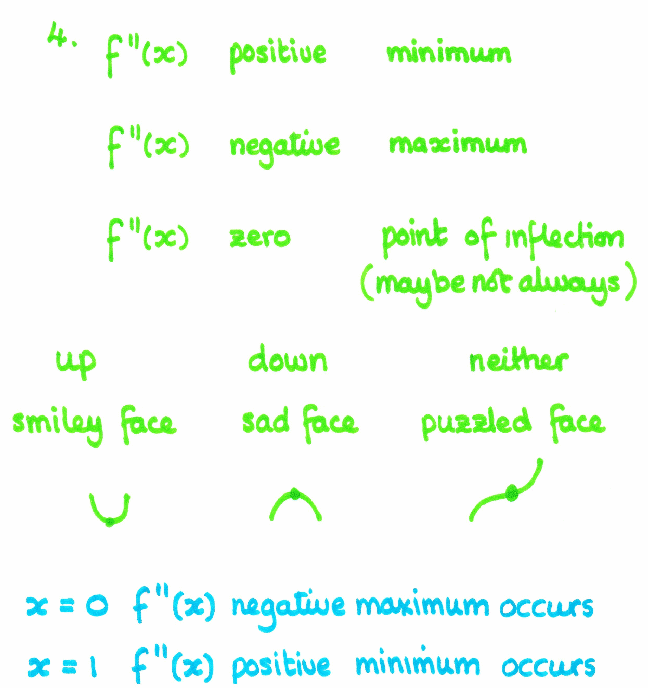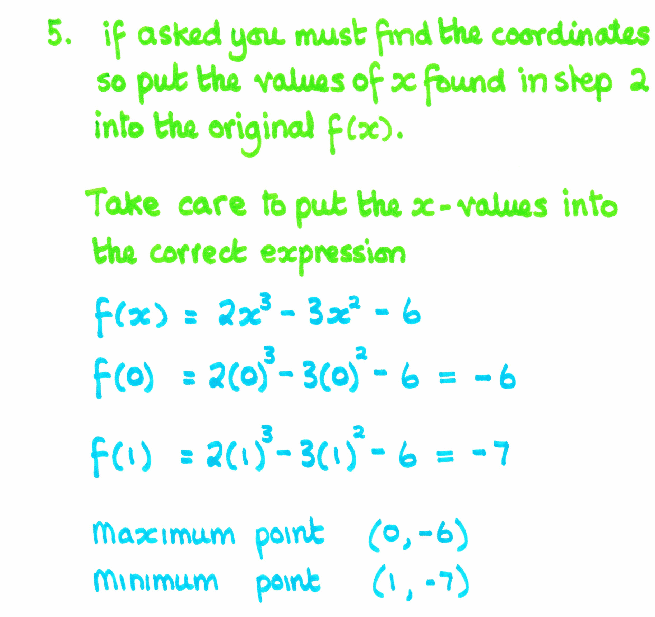Coming to Statistica
in 2022/2023

Group Classes

Interactive Webinars

Blog

Guestbook

.

E-mail        anne@statistica.com.au

# Place         Willetton, Western Australia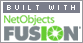# Mathematics & Statistic Tutor Perth - SPSS Help# Finding Maximum and Minimum valuesby Differentiation### Co-ordinates

When the question asks to find the co-ordinates, you will be expected to state both  x and y values.
It does not matter whether it is a maximum or a minimum or just a point on the curve, you will still have to state both values.
You will find the co-ordinates by substituting the values back into the original equation, f(x).

A co-ordinate is written (x , y)

### Increasing, Decreasing or Stationary

f '(x) is negative   the function is decreasing
f '(x) is zero           the function is stationary (not changing)
f '(x) is positive     the function is increasing.### Maximum, MinimumPoints of Inflection

The value f '(x) is the gradient at any point but often we want to find the Turning or Stationary Point (Maximum and Minimum points) or Point of Inflection
These happen where the gradient is zero,  f '(x) = 0.

Critical Points include Turning points and Points where f ' (x) does not exist.

f ''(x)  is negative   the function is maximum turning point
f ''(x) is zero            the function may be a point of inflection
f ''(x) is positive      the function is
minimum turning point

### Concave Up, Down or Neither

f ''(x) is negative    the function is concave downwards
f
''(x) is zero            the function changing from concave
downwards to upwards (or the other way around)
f
''(x) is positive      the function is
concave upwards.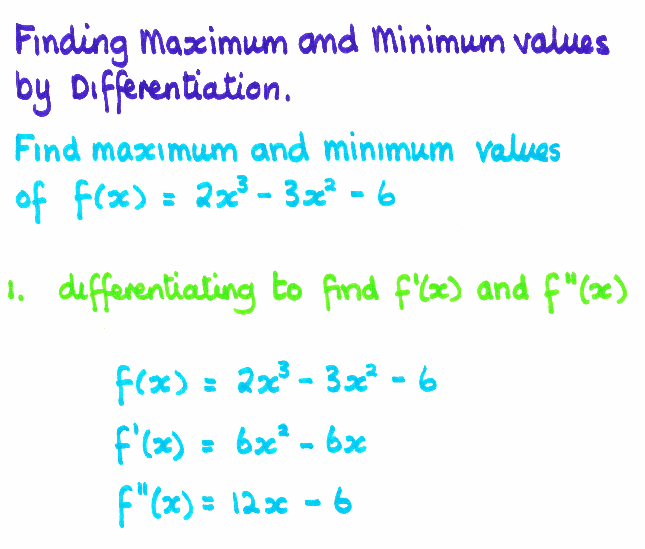#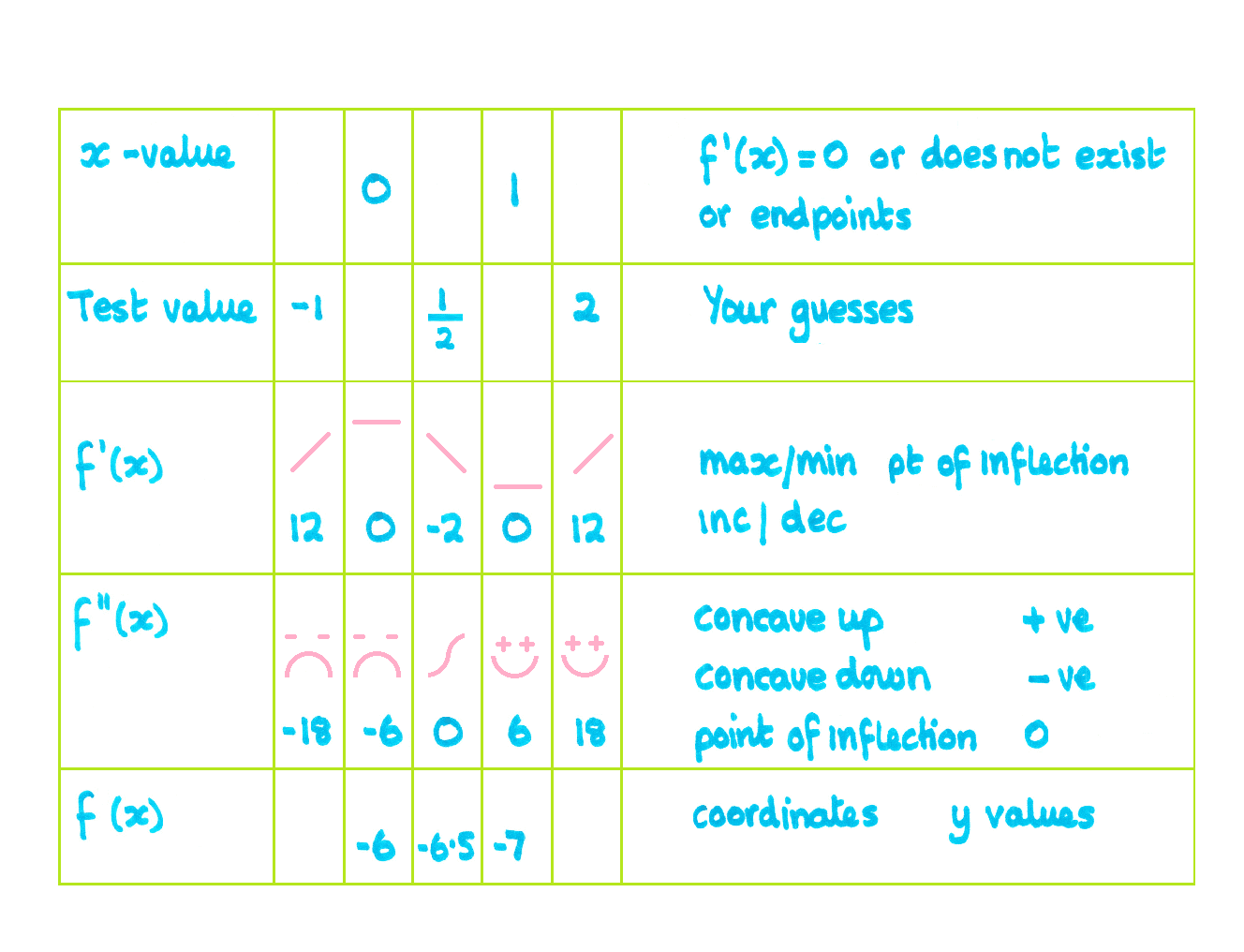Click here for instructions how to construct the tableto find Maximum, Minimum, Increasing and Decreasing intervals and Concavity